Notebook

# Quantpedia Series: Do Momentum and Reversals Coexist?¶

By Jeremy Muhia

This research is published in partnership with Quantpedia, an online resource for discovering new trading ideas.

You can view the full Quantpedia series in the library along with other research and strategies.

## Run this cell before proceeding¶

Whitepaper author(s): Jason Wei (wei@rotman.utoronto.ca)

Whitepaper source: https://papers.ssrn.com/sol3/papers2.cfm?abstract_id=1679464

### Abstract¶

From Quantpedia:

The answer to the title question is "Yes." Examining stocks traded on the NYSE, AMEX and NASDAQ for the period of 1964 to 2009, this study discovers that, while momentum prevails among small stocks, momentum and reversals coexist among large stocks for a holding period of up to six months. The momentum/reversal divide is along the volatility dimension: Large-cap/low-volatility stocks exhibit reversals while large-cap/high-volatility stocks experience momentum. This new discovery cannot befully rationalized with either risk-based or behavioral-based explanations.

As found in the paper, stocks of large firms displayed momentum and reversals. Large firm stocks with high volatility showed momentum when taking the mean of returns for all stocks in this category. That is, large firm stocks with high volatility had high rate of acceleration in their prices. Large firm stocks with low volatility, however, showed reversals. In other words, large firm stocks with low volatility had changes in the direction of their price trends.

### Introduction¶

Performing sequential sorts on size, volatility and past returns, this study discovers that, over a holding period of up to six months, momentum and reversals coexist for large stocks. Specifically, large-cap/high-volatility stocks experience momentum while large-cap/low-volatility stocks exhibit reversals. For large-cap/low-volatility stocks, a statistically significant reversal, instead of a weaker momentum as suggested by previous findings, is observed.

At the beginning of each month, realized returns and realized (annualized) volatilities are calculated for each stock for the past one, two, three, six and 12 months. One week (seven calendar days) prior to the beginning of each month is skipped to avoid biases due to microstructure issues (see, e.g., Jegadeesh and Titman, 1993; and Chan, Jegadeesh and Lakonishok, 1996).

Similar to the methodology of the original paper, a three way sort was used to construct portfolios. First, firms were divided along the median market cap. Firms with a market cap below the median were considered "small" and firms with a market cap above the median were considered "large". The market cap quantile of each stock was stored in the market_cap_quantiles column.

Next, annualized volatility with a 30 day window was calculated for each stock. These values were then grouped into 5 quantiles with the stocks at the lowest quantile receiving a value of 0 and stocks at the highest quantile receiving a value of 4. These volatility quantiles were stored in the volatility_quantiles column.

Finally, past returns were calculated. Delayed past returns were also calculated with a 7 day delay in order to minimize microstructure issues as in the paper. For each lookback window used to calculated past returns, stocks were grouped into 5 quantiles based on past delayed performance. These groupings of past performance were stored in the n day lookback - delayed columns.

The objective of this study was to implement this three step sorting method to create portfolios of large firms with low/high volatility and low/high returns in order to identify the reversal and momentum phenomena mentioned above.

You can navigate the rest of this notebook as follows:

# Methodology and Sample¶

For the purpose of this study, portfolios are constructed using the metrics listed above. First, we measure and rank the the stocks along market cap, volatility, and delayed returns. Then, for large firms, low volatility stocks are grouped together and high volatility stocks are grouped together. Finally, within these two groups, we calculate the mean returns of the lowest performing and highest performing stocks.

This is based on the idea that the within the low volatility group, the reversal effect will cause the prices of stocks in this group to reverse, a phenomena that can be taken advantage of.

On the other had, within the high volatility group, momentum will cause the prices of high performing stocks in this group to continue to increase, another phenomena that can be taken advantage of.

In :
data = create_data(START, END)
# start at beginning of 09 until end of 2016

Done with run_split_pipeline()
Done with get_pricing()
Calculating returns given hold times
Done calculating returns given hold time
Done with compute_returns()

Out:
day SID 10 day lookback 10 day lookback - delayed 11 day lookback 11 day lookback - delayed 12 day lookback 12 day lookback - delayed 13 day lookback 13 day lookback - delayed ... 21 day hold time 22 day hold time 23 day hold time 24 day hold time 25 day hold time 26 day hold time 27 day hold time 28 day hold time 29 day hold time 30 day hold time
0 2010-12-01 00:00:00+00:00 Equity(2 [ARNC]) 2 1 1 1 1 2 1 2 ... 0.138590 0.185018 0.232222 0.223984 0.252440 0.232222 0.251692 0.219491 0.227729 0.208178
1 2010-12-01 00:00:00+00:00 Equity(24 [AAPL]) 3 2 3 2 3 2 2 2 ... 0.024359 0.032903 0.054472 0.045291 0.061694 0.059368 0.074742 0.093911 0.088746 0.094817
2 2010-12-01 00:00:00+00:00 Equity(62 [ABT]) 0 0 0 0 0 0 0 0 ... 0.011712 0.029856 0.024523 0.028340 0.034301 0.026614 0.030065 0.027503 0.029281 0.028863
3 2010-12-01 00:00:00+00:00 Equity(67 [ADSK]) 2 2 2 2 2 2 1 2 ... 0.083707 0.081467 0.099664 0.105543 0.152296 0.159295 0.132139 0.152856 0.120101 0.131859
4 2010-12-01 00:00:00+00:00 Equity(76 [TAP]) 1 1 1 1 1 1 1 2 ... 0.045028 0.046709 0.037775 0.010591 0.006028 -0.004131 -0.016378 -0.017411 -0.003938 -0.008285

5 rows × 218 columns

In :
benchmark_returns = create_benchmark_returns()


### Quality Testing the Data¶

Below, we output the dimensions of the Dataframe in order to verify it against our approximations. Given that the Q1500 contains roughly 1500 stocks, we expect the number of rows to be roughly (1500 stocks each day x 252 days in a year x N years = number of rows).

Yet, because of constrains when doing computations with a large volume of data (in this case, a large universe of stocks evaluated over many years), we only create data once every 15 days. Also, because of events such as a stock delisting or being removed/added to the universe, the actual results may differ from our calculation.

In :
print(data.shape)
print(len(data['SID'].unique()))
print(len(data['day'].unique()))

(216394, 218)
2388
146


## Sample Statistics¶

### Mean Volatility at Each Quintile¶

Mirroring the methodology found in the whitepaper, volatility is classified using quintiles. As expected, the mean volatility at each quintile is increasing.

In :
fig, ax = plt.subplots()
mean_volatility = data.groupby('volatility_quantiles')['volatility'].mean()
graph = plt.bar(list(range(5)), mean_volatility, align='center')
ax.set(title='Mean Annualized Volatility by Volatility Quintile', ylabel='Mean Annualized Volatility', xlabel='Volatility Quintile')

Out:
[<matplotlib.text.Text at 0x7f7ddb328ad0>,
<matplotlib.text.Text at 0x7f7de1acaed0>,
<matplotlib.text.Text at 0x7f7dda4f3590>]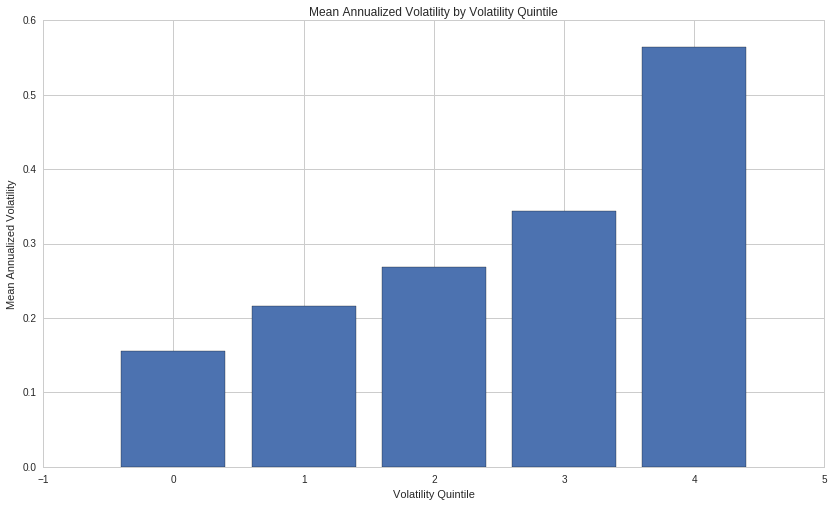### Summary Statistics¶

The visualization below digs deeper into the Pipeline's volatility. This visualization includes volatility at the median, 75th, and 25th percentiles at each volatility quintile. Outliers are also shown at each volatility quintile.

In :
grouped = data[['volatility', 'volatility_quantiles']]
graph = grouped.boxplot(by='volatility_quantiles')
graph.set(ylabel='Annualized Volatility', xlabel='Volatility Quantiles', title='Annualized Volatility Quantiles Statistics')
plt.suptitle('')

Out:
<matplotlib.text.Text at 0x7f7dd99bd5d0>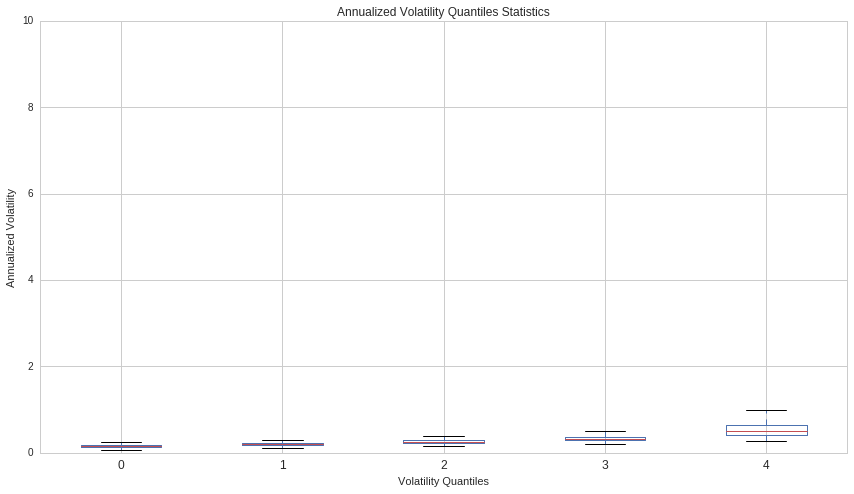### Average Dollar Volume vs. Volatility¶

In the graph below, Average Dollar Volume is plotted against Volatility for each security in the pipeline. As expected, low volatility is related to higher Average Dollar Volume. Frequently traded stocks are less likely to experience significant price changes, and therefore have lower volatility.

In :
fig, ax = plt.subplots()
x = data['volatility']

m, b = np.polyfit(x, y, 1)

plt.plot(x, y, '.')
plt.plot(x, m * x + b, '-')
ax.set(xlabel='Volatility', ylabel='Average Dollar Volume')

Out:
[<matplotlib.text.Text at 0x7f7dd9f70490>,
<matplotlib.text.Text at 0x7f7dd6ee9dd0>]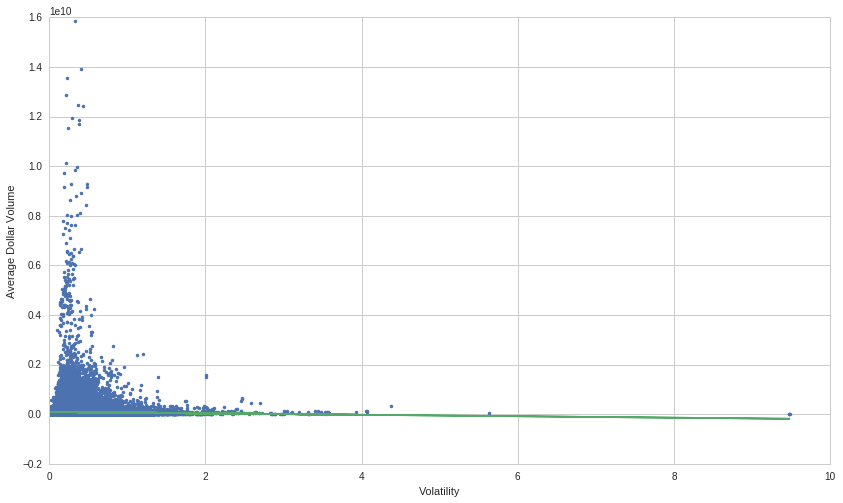# Results¶

In keeping with the methodology of the original study:

1. Divide the universe into 2 market cap groups using the median
• Large firms have a market cap above the median
• Small firms have a market cap below the median
2. Within the market cap buckets, group the stocks into 5 volatility quantiles
3. Calculate the mean returns of the large firms with the lowest and highest volatility
• The lookback periods are from 2 to 90 days inclusive, [2, 90]

## Comparing average return matrixes for past performers/past losers¶

Using this returns data, we observe that for large firms in the lowest volatility quintile, the portfolio of high performing stocks continues to outperform the portfolio of low performing stocks only when using a short term lookback period.

However, the results of the whitepaper remain true when using a longer term lookback period. Beyond a roughly 18 day lookback period, low performing stocks begin to outpace high performing stocks at the lowest volatility quantile.

In :
fig, ax = plt.subplots()
ax = sns.heatmap(create_spread_matrix_original(returns_data=data, returns_quantile=0, volatility_quantile=0, market_cap_decile=1), annot=True)
ax.set(
xlabel='Lookback Period (in Days)',
ylabel='Holding Period (in Days)',
title='Average Returns for Large Firms in the Lowest Returns Quintile (P1) and Lowest Volatility Quintile'
)
ax.plot()

Out:
[]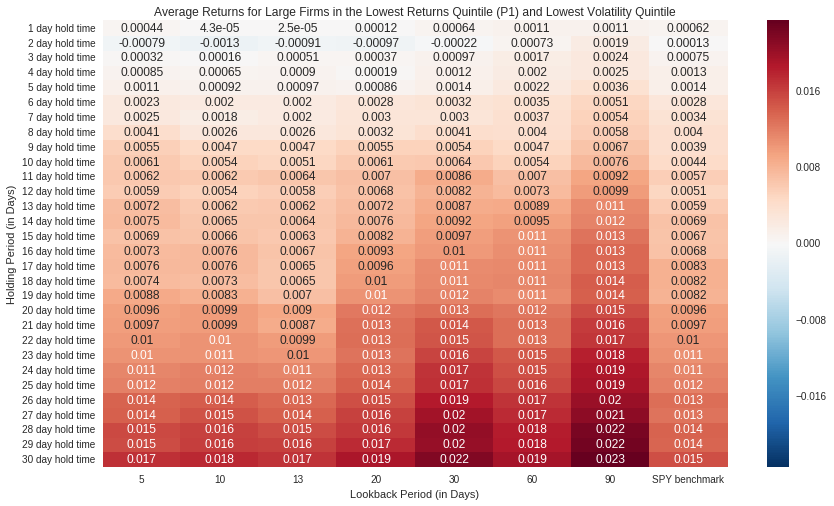In :
fig, ax = plt.subplots()
ax = sns.heatmap(create_spread_matrix_original(returns_data=data, returns_quantile=4, volatility_quantile=0, market_cap_decile=1), annot=True)
ax.set(
xlabel='Lookback Period (in Days)',
ylabel='Holding Period (in Days)',
title='Average Returns for Large Firms in the Highest Returns Quintile (P5) and Lowest Volatility Quintile'
)
ax.plot()

Out:
[]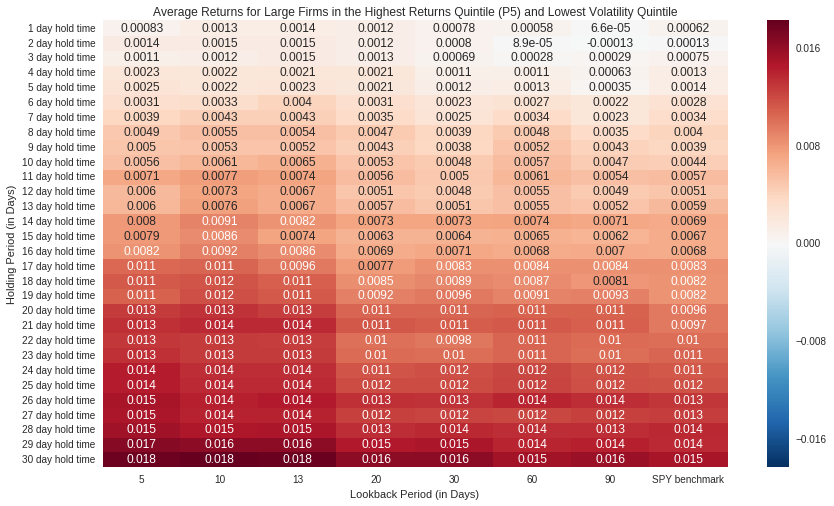### Summary¶

Above, the matricies show the mean returns for the worst and best performing stocks of large firms with low volatility. The paper suggests that for large firms with low volatility, we should expect a price reversal.

### Returns Spreads for Large firms at the Lowest Volatility Quantile¶

Below, we observe at a closer level what is demonstrated in the spread matricies above. That is, as the lookback period increases with a constant hold time of 30 days, returns spreads increase. This is consistent with what is observed in the whitepaper where increasing hold times and lookback periods result in historically low performing stocks to perform better at the lowest volatility quantile.

Curiously, the returns spread shrinks with a medium term lookback periods then increases again for long term lookback periods.

In :
plot_spreads_original(stock_data=data, market_cap_quantile=1, volatility_quantile=0, hold_time='30 day hold time')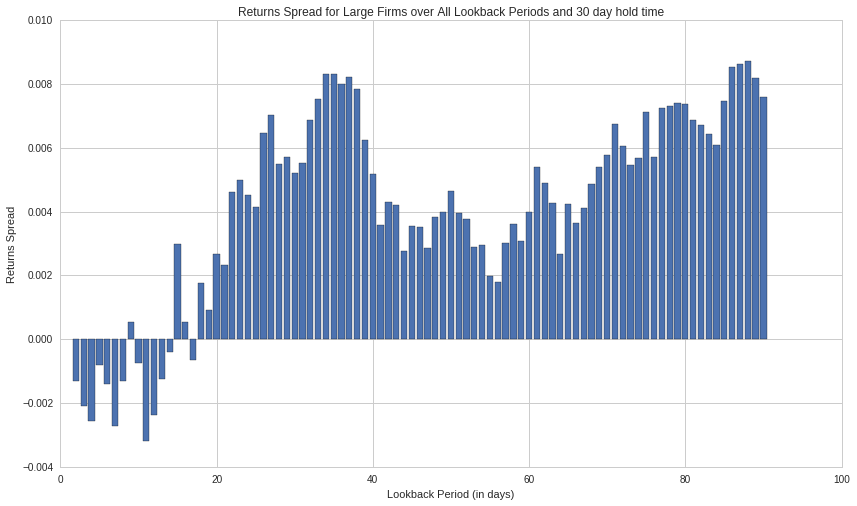This is maintained at a short term hold time as well.

So, as hold time increases, the mean returns of the lowest performing stocks continue to increase while the mean returns of the best performing stocks continue to decrease or stagnate. This aligns with the conclusion that for large firms with low volatility, a reversal affect occurs for previously high performing stocks and for previously low performing stocks.

Overall, we observe a change in the direction of the price trends. That change results in previously low performing stocks to generate higher returns than previously high performing stocks.

In :
plot_spreads_original(stock_data=data, market_cap_quantile=1, volatility_quantile=0, hold_time='1 day hold time')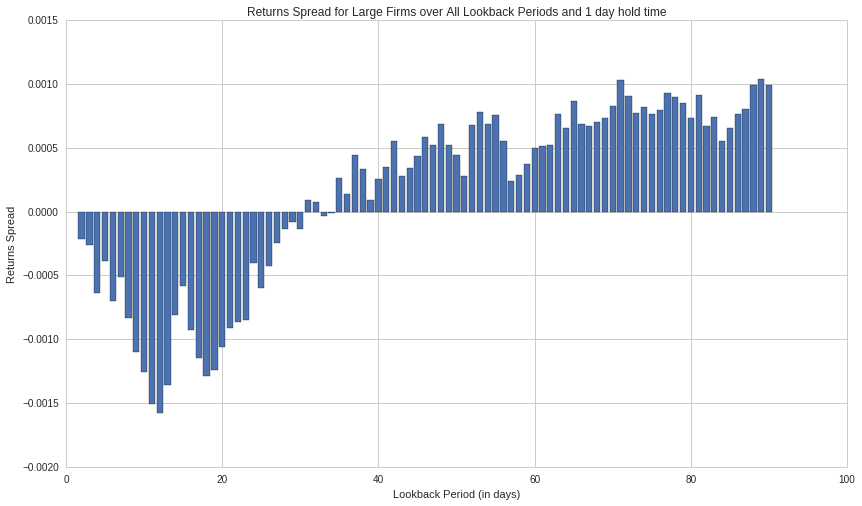## Comparing average return matrixes for past performers/past losers cont'd...¶

Now, we study the performance of this strategy at the highest volatility quintile.

Here, the results show that the portfolio of high performing stocks has greater returns than the portfolio of low performing stocks.

But, when using a short term lookback period, the portfolio of low performing stocks does continue to have greater returns than the portfolio of high perfoming stocks.

In :
fig, ax = plt.subplots()
ax = sns.heatmap(create_spread_matrix_original(returns_data=data, returns_quantile=0, volatility_quantile=4, market_cap_decile=1), annot=True)
ax.set(
xlabel='Lookback Period (in Days)',
ylabel='Holding Period (in Days)',
title='Average Returns for Large Firms in the Lowest Returns Quintile (P1) and Highest Volatility Quintile'
)
ax.plot()

Out:
[]